SNAP Library 6.0, Developer Reference  2020-12-09 16:24:20 SNAP, a general purpose, high performance system for analysis and manipulation of large networks
TNGraph::TEdgeI Class Reference

Edge iterator. Only forward iteration (operator++) is supported. More...

`#include <graph.h>`

Collaboration diagram for TNGraph::TEdgeI: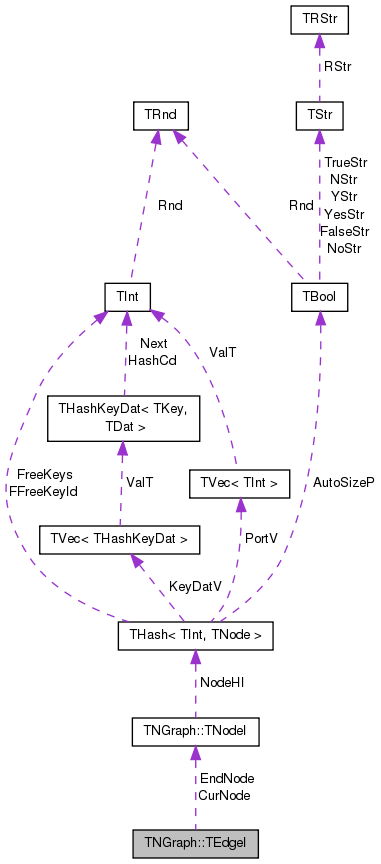[legend]

## Public Member Functions

TEdgeI ()

TEdgeI (const TNodeI &NodeI, const TNodeI &EndNodeI, const int &EdgeN=0)

TEdgeI (const TEdgeI &EdgeI)

TEdgeIoperator= (const TEdgeI &EdgeI)

TEdgeIoperator++ (int)
Increment iterator. More...

bool operator< (const TEdgeI &EdgeI) const

bool operator== (const TEdgeI &EdgeI) const

int GetId () const
Returns edge ID. Always returns -1 since only edges in multigraphs have explicit IDs. More...

int GetSrcNId () const
Returns the source node of the edge. More...

int GetDstNId () const
Returns the destination node of the edge. More...

TNodeI CurNode

TNodeI EndNode

int CurEdge

class TNGraph

## Detailed Description

Edge iterator. Only forward iteration (operator++) is supported.

Definition at line 430 of file graph.h.

## Constructor & Destructor Documentation

 TNGraph::TEdgeI::TEdgeI ( )
inline

Definition at line 435 of file graph.h.

435 : CurNode(), EndNode(), CurEdge(0) { }
TNodeI CurNode
Definition: graph.h:432
TNodeI EndNode
Definition: graph.h:432
 TNGraph::TEdgeI::TEdgeI ( const TNodeI & NodeI, const TNodeI & EndNodeI, const int & EdgeN = `0` )
inline

Definition at line 436 of file graph.h.

436 : CurNode(NodeI), EndNode(EndNodeI), CurEdge(EdgeN) { }
TNodeI CurNode
Definition: graph.h:432
TNodeI EndNode
Definition: graph.h:432
 TNGraph::TEdgeI::TEdgeI ( const TEdgeI & EdgeI )
inline

Definition at line 437 of file graph.h.

437 : CurNode(EdgeI.CurNode), EndNode(EdgeI.EndNode), CurEdge(EdgeI.CurEdge) { }
TNodeI CurNode
Definition: graph.h:432
TNodeI EndNode
Definition: graph.h:432

## Member Function Documentation

 int TNGraph::TEdgeI::GetDstNId ( ) const
inline

Returns the destination node of the edge.

Definition at line 449 of file graph.h.

References TNGraph::TNodeI::GetOutNId().

449 { return CurNode.GetOutNId(CurEdge); }
TNodeI CurNode
Definition: graph.h:432
int GetOutNId(const int &NodeN) const
Returns ID of NodeN-th out-node (the node the current node points to).
Definition: graph.h:416

Here is the call graph for this function: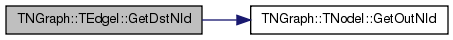Here is the caller graph for this function: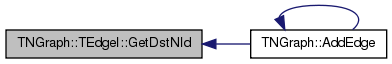int TNGraph::TEdgeI::GetId ( ) const
inline

Returns edge ID. Always returns -1 since only edges in multigraphs have explicit IDs.

Definition at line 445 of file graph.h.

445 { return -1; }
 int TNGraph::TEdgeI::GetSrcNId ( ) const
inline

Returns the source node of the edge.

Definition at line 447 of file graph.h.

References TNGraph::TNodeI::GetId().

447 { return CurNode.GetId(); }
TNodeI CurNode
Definition: graph.h:432
int GetId() const
Returns ID of the current node.
Definition: graph.h:400

Here is the call graph for this function: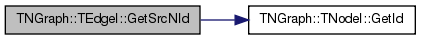Here is the caller graph for this function: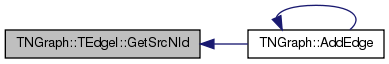TEdgeI& TNGraph::TEdgeI::operator++ ( int )
inline

Increment iterator.

Definition at line 440 of file graph.h.

References TNGraph::TNodeI::GetOutDeg().

440  { CurEdge++; if (CurEdge >= CurNode.GetOutDeg()) { CurEdge=0; CurNode++;
441  while (CurNode < EndNode && CurNode.GetOutDeg()==0) { CurNode++; } } return *this; }
TNodeI CurNode
Definition: graph.h:432
TNodeI EndNode
Definition: graph.h:432
int GetOutDeg() const
Returns out-degree of the current node.
Definition: graph.h:406

Here is the call graph for this function: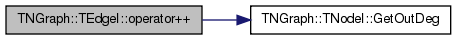bool TNGraph::TEdgeI::operator< ( const TEdgeI & EdgeI ) const
inline

Definition at line 442 of file graph.h.

References CurEdge, and CurNode.

442 { return CurNode<EdgeI.CurNode || (CurNode==EdgeI.CurNode && CurEdge<EdgeI.CurEdge); }
TNodeI CurNode
Definition: graph.h:432
 TEdgeI& TNGraph::TEdgeI::operator= ( const TEdgeI & EdgeI )
inline

Definition at line 438 of file graph.h.

References CurEdge, CurNode, and EndNode.

438 { if (this!=&EdgeI) { CurNode=EdgeI.CurNode; EndNode=EdgeI.EndNode; CurEdge=EdgeI.CurEdge; } return *this; }
TNodeI CurNode
Definition: graph.h:432
TNodeI EndNode
Definition: graph.h:432
 bool TNGraph::TEdgeI::operator== ( const TEdgeI & EdgeI ) const
inline

Definition at line 443 of file graph.h.

References CurEdge, and CurNode.

443 { return CurNode == EdgeI.CurNode && CurEdge == EdgeI.CurEdge; }
TNodeI CurNode
Definition: graph.h:432

## Friends And Related Function Documentation

 friend class TNGraph
friend

Definition at line 450 of file graph.h.

## Member Data Documentation

 int TNGraph::TEdgeI::CurEdge
private

Definition at line 433 of file graph.h.

Referenced by operator<(), operator=(), and operator==().

 TNodeI TNGraph::TEdgeI::CurNode
private

Definition at line 432 of file graph.h.

Referenced by operator<(), operator=(), and operator==().

 TNodeI TNGraph::TEdgeI::EndNode
private

Definition at line 432 of file graph.h.

Referenced by operator=().

The documentation for this class was generated from the following file: